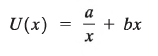# Problem: A particle of mass m moves under the influence of a potential energy large{U(x) = frac{a}{x} + bx}, where a and b are positive constants and the particle is restricted to the region x &gt; 0. Find a point of equilibrium for the particle and demonstrate that it is stable.

###### FREE Expert Solution

In this problem, we'll use the relationship between equilibrium and potential energy to determine the equilibrium point.

Force:

79% (276 ratings)###### Problem Details

A particle of mass m moves under the influence of a potential energy , where a and b are positive constants and the particle is restricted to the region x > 0. Find a point of equilibrium for the particle and demonstrate that it is stable.